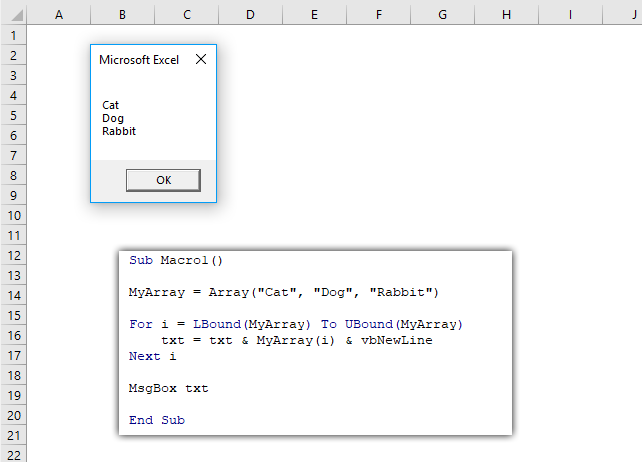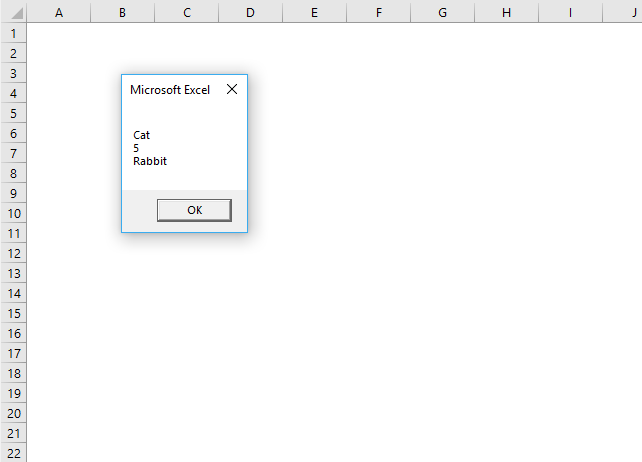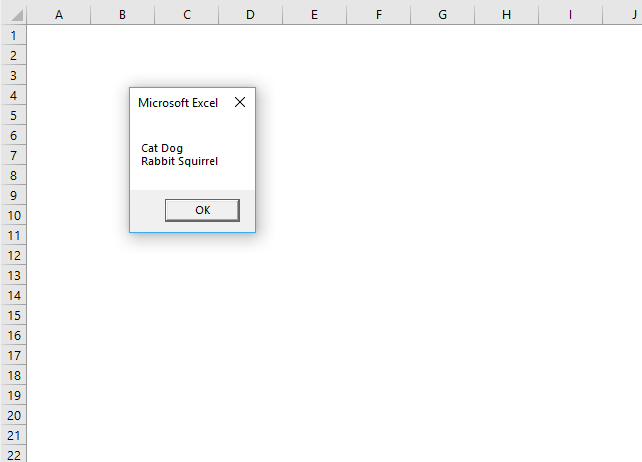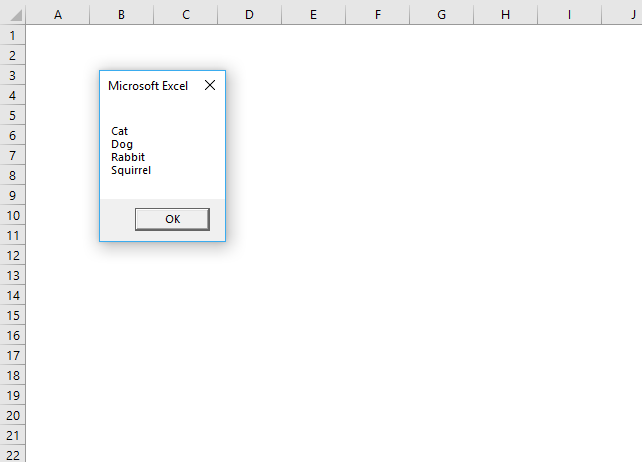Author: Oscar Cronquist Article last updated on October 24, 2018The ARRAY function creates a Variant variable containing array values.

```Sub Macro1()

MyArray = Array("Cat", "Dog", "Rabbit")

For i = LBound(MyArray) To UBound(MyArray)
txt = txt & MyArray(i) & vbNewLine
Next i

MsgBox txt

End Sub
```

The macro above populates variable MyArray with values Cat, Dog and Rabbit.

A FOR ... NEXT statement loops through the array variable using the Lbound and Ubound function to determine the lower and upper limit of the array.

An ampersand then concatenates the values and lastly, the msgbox shows the concatenated string.

Array(arglist)

### Arguments

 arglist A list of values. Text strings must have a beginning and ending double quote. The values must be separated by a comma. If omitted a zero-length array is created.

The following macro demonstrates another way to create an array variable. [arglist] means evaluate.```Sub Macro2()

MyArray = [{"Cat", 5, "Rabbit"}]

For i = LBound(MyArray) To UBound(MyArray)
txt = txt & MyArray(i) & vbNewLine
Next i

MsgBox txt

End Sub
```

This macro creates array variable MyArray with values in two dimensions.```Sub Macro3()

MyArray = Array(Array("Cat", "Dog"), Array("Rabbit", "Squirrel"))

For r = 0 To 1
For c = 0 To 1
txt = txt & MyArray(r)(c) & " "
Next c
txt = txt & vbNewLine
Next r

MsgBox txt

End Sub
```

This macro populates array variable MyArray with values in two dimensions using the evaluate characters.```Sub Macro4()

MyArray = [{"Cat", "Dog"; "Rabbit", "Squirrel"}]

For r = LBound(MyArray, 1) To UBound(MyArray, 1)
For c = LBound(MyArray, 2) To UBound(MyArray, 2)
txt = txt & MyArray(r, c) & vbNewLine
Next c
Next r

MsgBox txt

End Sub
```